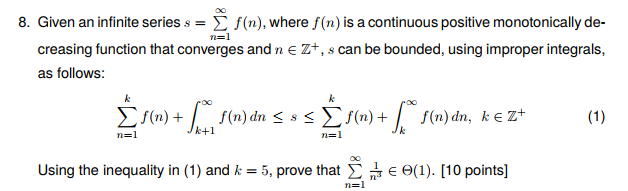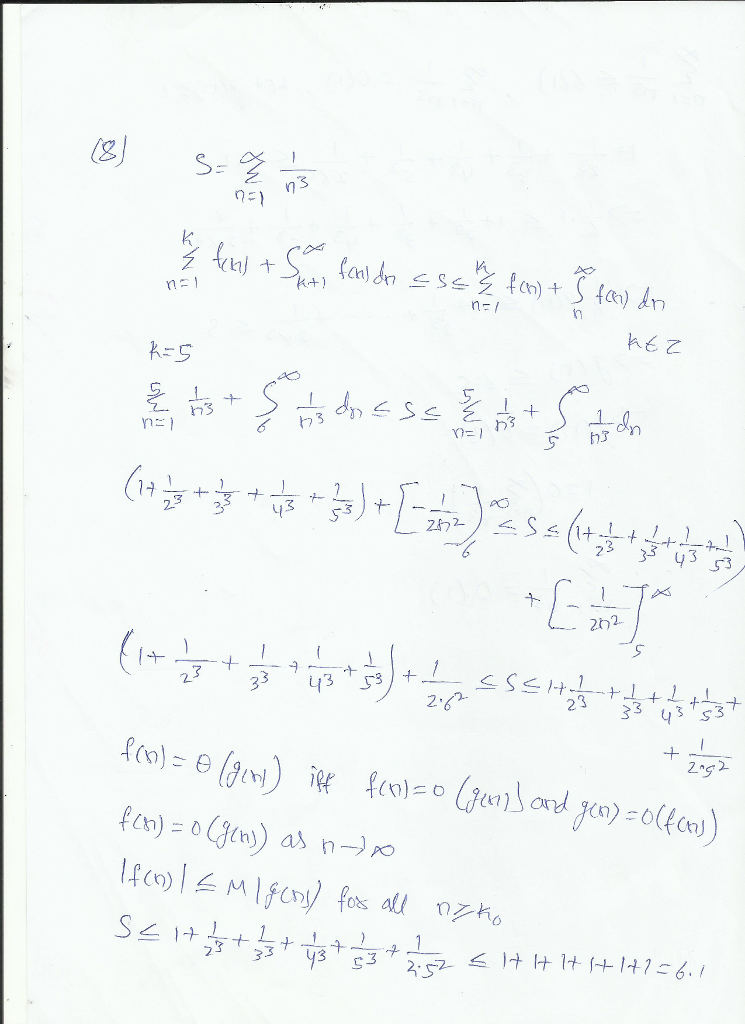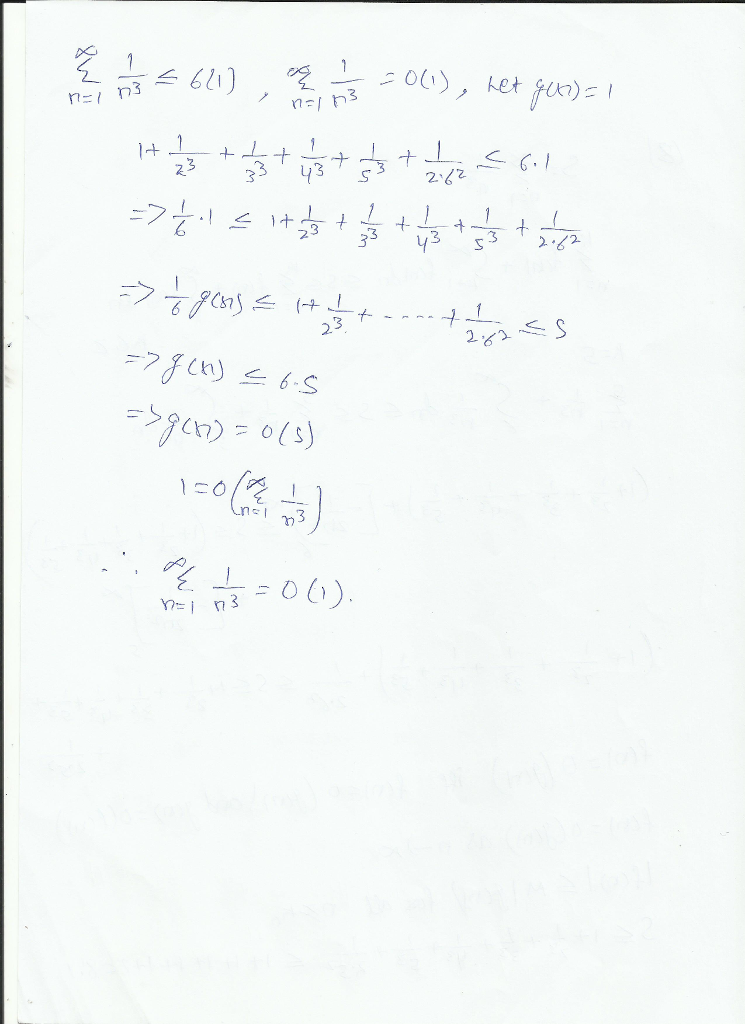# Homework Solution: Given an infinite series s = sigma^infinity _n=1 f(n), where f(n) is a continuous positive mon…Given an infinite series s = sigma^infinity _n=1 f(n), where f(n) is a continuous positive monotonically decreasing function that converges and n elementof Z^+, s can be bounded, using improper integrals, as follows: sigma^k _n=1 f(n) + integral^infinity _k+1 f(n) dn lessthanorequalto s lessthanorequalto sigma^k _n=1 f(n) + integral^infinity _k f(n) dn, k elementof Z^+ Using the inequality in (1) and k = 5, prove that sigma^infinity _n=1 1/n^3 elementof theta (1).